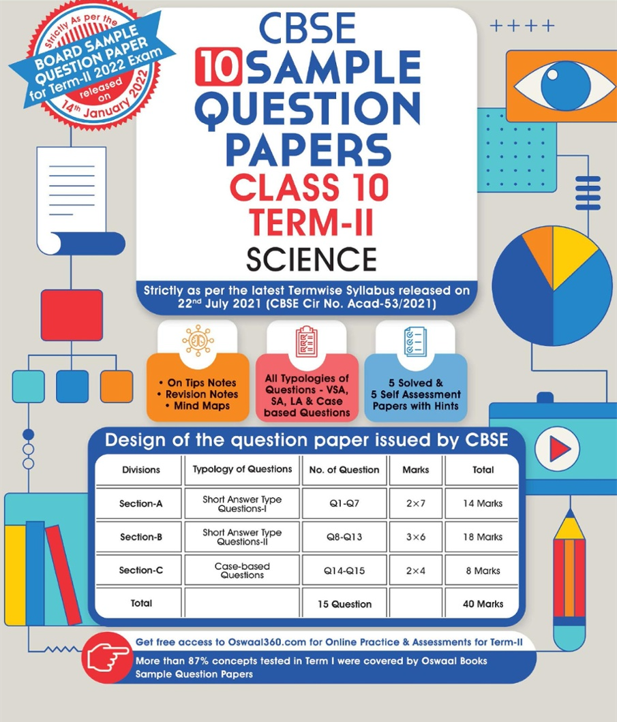# In the above circuit, if the current reading in the ammeter A is 2A, what would be the value of R1?

CBSE Sample Question Paper, Class 10 Science Term 2 Question 1 - (In the above circuit, if the current reading in the ammeter A is 2A, what would be the value of R1?)